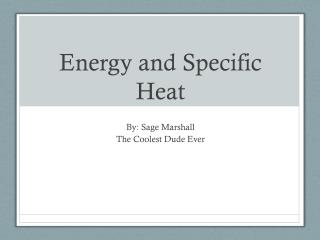DownloadDownload PresentationEnergy and Specific Heat

# Energy and Specific Heat

Download Presentation## Energy and Specific Heat

- - - - - - - - - - - - - - - - - - - - - - - - - - - E N D - - - - - - - - - - - - - - - - - - - - - - - - - - -
##### Presentation Transcript

1. Energy and Specific Heat By: Sage Marshall The Coolest Dude Ever

2. Heat Heat – energy due to temperature Measured in -Joules (J) -Calories (cal)

3. Heat -Heat flows between objects -Temperature is a property of objects

4. 1st Law of Thermodynamics Energy can’t be created or destroyed During any reaction, the total final energy is the same as the total initial energy

5. 2nd Law of Thermodynamics Energy flows from a higher temperature to a lower temperature

6. Specific Heat Let’s think back to the example with wood and hot metal. -If I input the same amount of energy, will both substances heat up to the same final temperature?

7. Specific Heat

8. Specific Heat The amount of energy it takes to raise 1g of material 1oC in temperature Variable: c Units:

9. The Heat Equation E = Heat energy m = mass c = specific heat ΔT = temperature change

10. Example 1 How much energy is needed to raise 10g of steel from 25oC to 30oC? (The specific heat of steel is 0.470J/gC).

11. Example 2 How much energy is needed to raise 10g of wood from 25oC to 30oC? (The specific heat of wood is 2.500J/gC).

12. Example 3 On a sunny day, each square centimeter of the ocean absorbs 180 Joules of energy from the sun each hour. Assume all the heat is absorbed in the first 10m of water, which has a mass of 1000g. How much does the water temperature increase? (The specific heat of water is 4.184J/gC.

13. Example 4 A 12.6g piece of zinc was heated to 99.5oC. It was then added to a beaker with cooler water. When the water and metal came to thermal equilibrium, the temperature of the zinc had dropped to 25.8oC. The zinc transferred 586J of energy to the water. What is the specific heat of zinc?